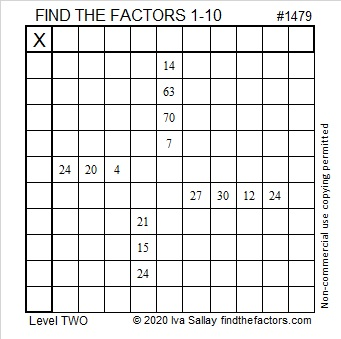# 1479 and Level 2

### Today’s Puzzle:

Can you find the factors from 1 to 10 that will work with the clues in this puzzle to make a multiplication table?### Factors of 1479:

• 1479 is a composite number.
• Prime factorization: 1479 = 3 × 17 × 29
• 1479 has no exponents greater than 1 in its prime factorization, so √1479 cannot be simplified.
• The exponents in the prime factorization are 1, 1, and 1. Adding one to each exponent and multiplying we get (1 + 1)(1 + 1)(1 + 1) = 2 × 2 × 2 = 8. Therefore 1479 has exactly 8 factors.
• The factors of 1479 are outlined with their factor pair partners in the graphic below.### Another Fact about the Number 1479:

1479 is the hypotenuse of FOUR Pythagorean triples:
396-1425-1479 which is 3 times (132-475-493)
465-1404-1479 which is 3 times (155-468-493)
696-1305-1479 which is (8-15-17) times 87
1020-1071-1479 which is (20-21-29) times 51